# On Euler’s And Milne’s Linear Multi Step Methods of Solving the Ordinary Differential Equations [2010MSC: 65XX]

## Abstract

This work discusses the Euler’s and Milne’s linear multistep methods for solving initial value problems of the ordinary differential equations. By this for the Euler’s we seek an approximation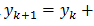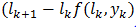and for the integral equation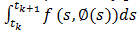with a truncation error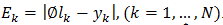. Thereby providing an enhancement accuracy and for the Milne’s method for the integral equation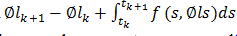the iterative formula: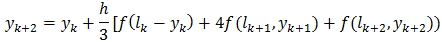Converges faster where the local truncation error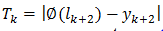is sufficiently negligible compared to that of Euler. Hence, the milne’ method is a more accurate approximation method than the Euler’s method. Section three points to the fact that linear multi step method so far discussed is convergent provided it is consistent and stable. This result is achievable if the root conditions are satisfied.

Keywords:
Continuous functions, ordinary differential equations iterations, integral equations, initial value problems, convergence

## Article Details

How to Cite
Emmanuel, E. C., & Roseline, O. N. (2020). On Euler’s And Milne’s Linear Multi Step Methods of Solving the Ordinary Differential Equations [2010MSC: 65XX]. Asian Journal of Pure and Applied Mathematics, 2(2), 1-18. Retrieved from https://globalpresshub.com/index.php/AJPAM/article/view/846
Section
Review Article

## References

Anderson KE. An introduction to numerical analysis. John Wiley and sons, New York. 1981;6:289-381.

Bulirsch R, Stoer J. Numerical treatment of ordinary differential equations by extrapolations number math. 1966;8:1–13.

Ceschino F, anf J. Kantzman. Numerical solution of the initial value problems. Prentice Hall Englewood Cliffs. N.J; 1966.

Collatz L. The numerical treatment of differential equations. 3rd Edition, Springer – Verlag, New York; 1966.

Dalquist G. Numerical integration of ordinary differential equations. Math. Scandinavia. 1956;4:33– 50.

Enright W, Hull T. Test results on initial value methods for non-stiff ordinary differential equations SIAMJ. Number. Anal. 1976;13:944-961.

Gear CW. Numerical initial value problems in ordinary differential equations. Prentice – Hall. Englewood Cliffs. N.J; 1971.

Isaacson E, Keller H. Analysis of numerical methods. John Willey, New York; 1966.

Lambert J. Computational methods in ordinary differential equations. John Wiley, New York; 1973.

Raltson A. A first course in numerical analysis. Mc Graw – Hill New York; 1966.